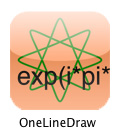English | Japanese

#OneLineDraw (iPad Application)

### What's new?

(2010.5.26) Available on iTunes App Store now! Free!

### Overview

Draw variety of figures by writing one line mathematical formula. With over 20 examples, you can enjoy this app even if you have no mathematical knowledge. Formulas you have written can be saved on your iPad, and can be saved as text files by iTunes' file sharing.

### Screen Shots

(Click to enlarge)

### How to write your own formula?

The screen is a complex plane of the range [-1,1] of real and imaginary part. You can use the following operator/functions. Your formula must be in 120 characters max.

NotationTypeDescription
x - ybinary operator Subtraction
x * ybinary operator Multiplication
x / ybinary operator Division
+ xunary operator (do nothing)
- xunary operator Minus
tvariable Parameter sweeping from 0 to 1
iconstant Imaginary Unit
econstant Napier's constant
piconstant Circumference ratio
real(z)function Real part
imag(z)function Imaginary part
abs(z)function Absolute value
arg(z)function Argument
conj(z)function Conjugate
sqrt(z)function Square root
exp(z)function Exponent (ez)
pow(x,y)function Power (xy)
log(z)function Natural logarithm (logez)
log10(z)function Common logarithm (log10z)
sin(z)function Sine
cos(z)function Cosine
tan(z)function Tangent
sinh(z)function Hyperbolic Sine
cosh(z)function Hyperbolic Cosine
tanh(z)function Hyperbolic Tangent

### Support

Email: okayan (at) c-creators.co.jp